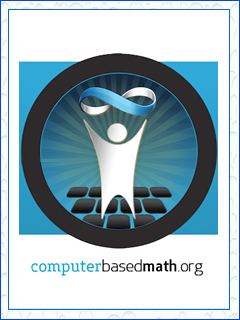# Computer based activities#### Activities at computerbasedmaths.org

Computer based mathematics activities for teachers, developed by the Wolfram team. Students will be engaged with the real world, a key part of which is using computers for doing most of the calculations.

#### Questions

Sample questions to work through with your students

• How long should my password be?
• Where is the reflection?
• Are the digits of π truly random?

#### Teaching kids real maths with computers

Conrad Wolfram's presents "Teaching Kids Real Maths with Computers". Conrad Wolfram says that part of maths we teach – calculation by hand – is mostly irrelevant to real mathematics and the real world. He argues for teaching kids maths through computer programming.

#### First Computer Based Maths country

developed with DB Informatics